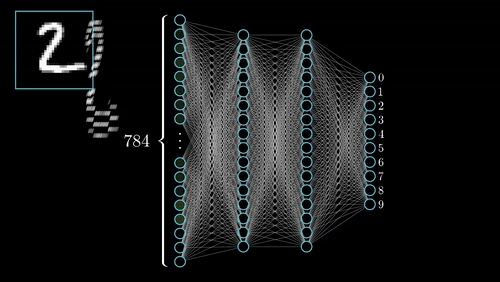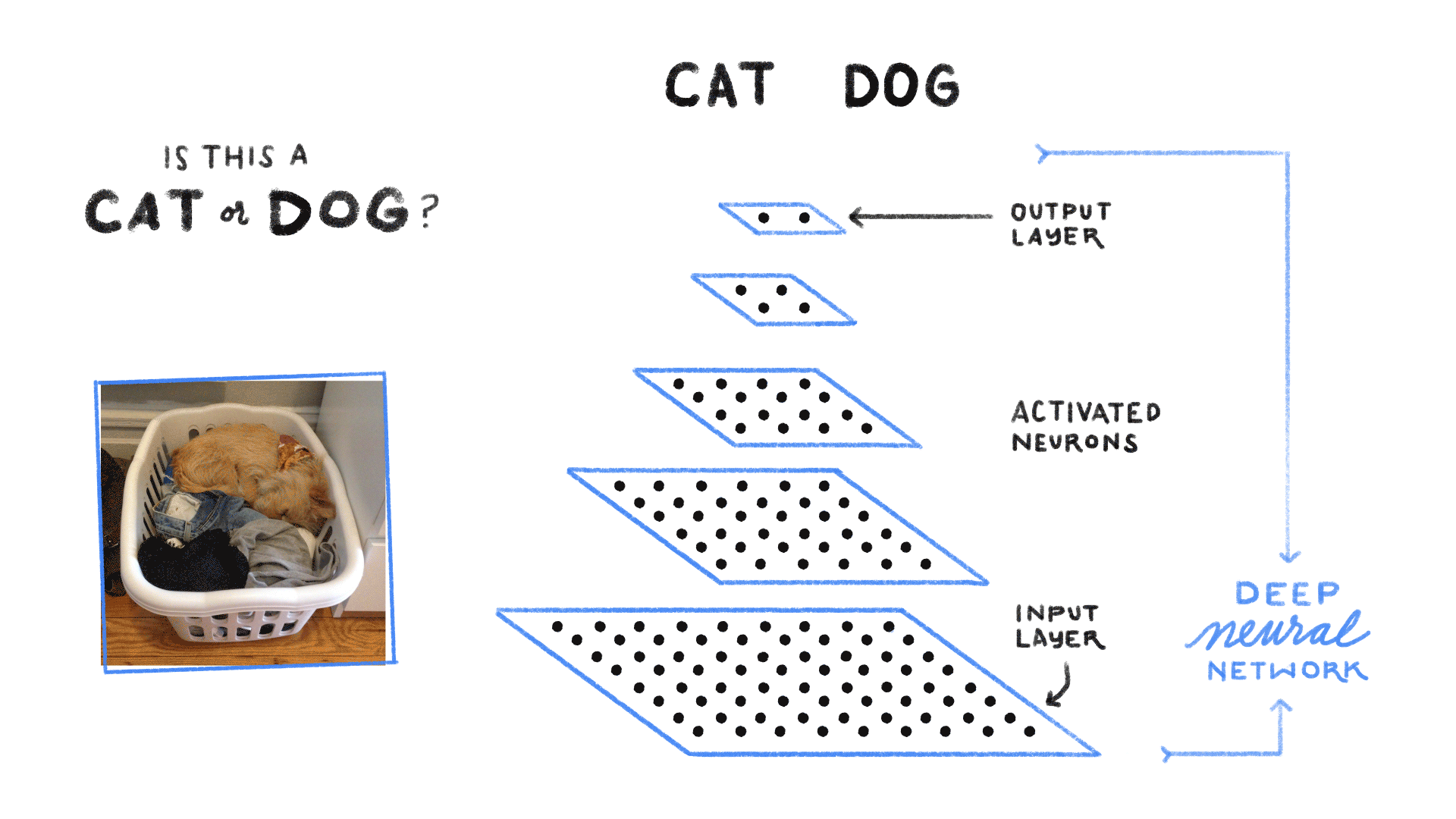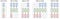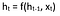Recurrent Neural Networks and LSTM explained

In this post we are going to explore RNN’s and LSTM

Recurrent Neural Networks are the first of its kind State of the Art algorithms that can Memorize/remember previous inputs in memory, When a huge set of Sequential data is given to it.

Before we dig into details of Recurrent Neural networks, if you are a Beginner i suggest you to read A Beginner intro to Neural Networks and A Beginner intro to Convolutional Neural Networks.

Now in this, we will learn:

• what Neural Networks do?
• why not Neural Networks/Feed forward Networks?
• Why/what are Recurrent Neural Networks?
• Different Types of RNN’s
• Deep view into RNN’s
• Character level language model
• Back propogation through time(BTT)
• Issues of RNN’s?
• Why LSTM’s?
1. Forget gate
2. input gate
3. output gate.
• Resources

What they do ?

ANNs, like people,like child, they even learn by example. An ANN is configured for a specific application, such as pattern recognition or data classification,Image recognition, voice recognition through a learning process.

Neural networks (NN) are universal function approximaters so that means neural networks can learn an approximation of any function f() such that.

y = f(x)NN trying to predict the image(data) that given to it. it predicts that the no is 2 hereA Neural network/feed forward Neural network predicting cat image

Why Not Neural Networks/Feed forward Networks?

In this training process, the first picture that the Neural network exposed to, will not necessarly alter how it classifies the Second one.

here the output of Cat does not relate to the output Dog. There are several scenario’s where the previous understanding of data is important.for example: Reading book, understanding lyrics,..,. These networks do not have memory in order to understand Sequential data like Reading books.

how do we overcome this challenge of understanding previous output?

solution: RNN’s.

What are RNN’s?

“Whenever there is a sequence of data and that temporal dynamics that connects the data is more important than the spatial content of each individual frame.”

– Lex Fridman (MIT)

Different types of RNN’sDifferent types of Recurrent Neural Networks. (2) Sequence output (e.g. image captioning takes an image and outputs a sentence of words). (3) Sequence input (e.g. sentiment analysis where a given sentence is classified as expressing positive or negative sentiment). (4) Sequence input and sequence output (e.g. Machine Translation: an RNN reads a sentence in English and then outputs a sentence in French). (5) Synced sequence input and output (e.g. video classification where we wish to label each frame of the video). Notice that in every case are no pre-specified constraints on the lengths sequences because the recurrent transformation (green) is fixed and can be applied as many times as we like.

Each rectangle in above image represent Vectors and Arrows represent functions. Input vectors are Red in color, output vectors are blue and green holds RNN’s state.

One-to-one:

Ex: Image classification.

One-to-Many:

Ex:Image Captioning takes image as input and outputs a sentence of words.

Many-to-One:

Ex:sentiment analysis where a given sentence is classified as expressing positive or negative sentiment.

Many-to-Many:

Ex: Machine Translation, where an RNN reads a sentence in English and then outputs a sentence in French.

Bidirectional Many-to-Many:

Ex: video classification where we wish to label each frame of the video.

Deep view into RNN’s:

where Hidden layer in RNN’s have same weights and bias through out the process giving them the chance to memorize information processed through them.

Current time stamp:

look at the above figure, where the formula for the Current state:function using previous times stamp and input gives current time stamp

where Ot is output state, ht current time stamp, ht-1 → is previous time stamp, and xt is passed as input state.

Applying activation function:

W is weight, h is the single hidden vector, Whh is the weight at previous hidden state, Whx is the weight at current input state.

Where tanh is the Activation funtion, that implements a Non-linearity that squashes the activations to the range[-1.1]

Output:

Yt is the output state. Why is the weight at the output state.

Example: “Character level language model” explained below.

Character level language model:

As a working example, suppose we only had a vocabulary of four possible letters “helo”, and wanted to train an RNN on the training sequence “hello”. This training sequence is in fact a source of 4 separate training examples: 1. The probability of “e” should be likely given the context of “h”, 2. “l” should be likely in the context of “he”, 3. “l” should also be likely given the context of “hel”, and finally 4. “o” should be likely given the context of “hell”.

Backpropogate Through Time:

We typically treat the full sequence (word) as one training example, so the total error is just the sum of the errors at each time step (character). The weights as we can see are the same at each time step. Let’s summarize the steps for backpropagation through time

1. The cross entropy error is first computed using the current output and the actual output
2. Remember that the network is unrolled for all the time steps
3. For the unrolled network, the gradient is calculated for each time step with respect to the weight parameter
4. Now that the weight is the same for all the time steps the gradients can be combined together for all time steps
5. The weights are then updated for both recurrent neuron and the dense layers

Note: Going back into every time stamp and updating its weights is really a slow process. It takes both the computational power and time.

While Backpropogating you may get 2 types of issues.

where the contribution from the earlier steps becomes insignificant in the gradient descent step.

While you are using Backpropogating through time, you find Error is the difference of Actual and Predicted model. Now what if the partial derivation of error with respect to weight is very less than 1?

If the partial derivation of Error is less than 1, then when it get multiplied with the Learning rate which is also very less. then Multiplying learning rate with partial derivation of Error wont be a big change when compared with previous iteration.

For ex:- Lets say the value decreased like 0.863 →0.532 →0.356 →0.192 →0.117 →0.086 →0.023 →0.019..

you can see that there is no much change in last 3 iterations. This Vanishing of Gradience is called Vanishing Gradience.

Aslo this Vanishing gradient problem results in long-term dependencies being ignored during training.

you Can Visualize this Vanishing gradient problem at real time here.

Several solutions to the vanishing gradient problem have been proposed over the years. The most popular are the aforementioned LSTM and GRU units, but this is still an area of active research.

similarly here, What if the Partial derivation of Errror is more than 1? Think.

How can you overcome the Challenges of Vanishing and Exploding Gradience?

1. Vanishing Gradience can be overcome with
• Relu activation function.
• LSTM, GRU.

2. Exploding Gradience can be overcome with

• Truncated BTT(instead starting backprop at the last time stamp, we can choose similar time stamp, which is just before it.)
• RMSprop to adjust learning rate.

1. Recurrent neural network are even used with convolutional layers to extend the effective pixel neighborhood.

1. Gradient vanishing and exploding problems.
2. Training an RNN is a very difficult task.
3. It cannot process very long sequences if using tanh or relu as an activation function.

Long Short Term Memory:

A special kind of RNN’s, capable of Learning Long-term dependencies.

LSTM’s have a Nature of Remembering information for a long periods of time is their Default behaviour.

LSTM had a three step Process:

look at the below figure that says Every LSTM module will have 3 gates named as Forget gate, Input gate, Output gate.

Forget Gate:

Decides how much of the past you should remember.

This gate Decides which information to be omitted in from the cell in that particular time stamp. It is decided by the sigmoid function. it looks at the previous state(ht-1) and the content input(Xt) and outputs a number between 0(omit this)and 1(keep this)for each number in the cell state Ct−1.

EX: lets say ht-1 →Roufa and Manoj plays well in basket ball.

Xt →Manoj is really good at webdesigning.

• Forget gate realizes that there might be change in the context after encounter its first fullstop.
• Compare with Current Input Xt.
• Its important to know that next sentence, talks about Manoj. so information about Roufa is omited.

Update Gate/input gate:

Decides how much of this unit is added to the current state.

Sigmoid function decides which values to let through 0,1. and tanh function gives weightage to the values which are passed deciding their level of importance ranging from-1 to 1.

EX: Manoj good webdesigining, yesterday he told me that he is a university topper.

• input gate analysis the important information.
• Manoj good webdesigining, he is university topper is important.
• yesterday he told me that is not important, hence forgotten.

Output Gate:

Decides which part of the current cell makes it to the output.

Sigmoid function decides which values to let through 0,1. and tanh function gives weightage to the values which are passed deciding their level of importance ranging from-1 to 1 and multiplied with output of Sigmoid.

EX: Manoj good webdesigining, he is university topper so the Merit student _______________ was awarded University Gold medalist.

• there could be lot of choices for the empty dash. this final gate replaces it with Manoj.

A Blog on LSTM’s with Nice visualization is here.

Resources:

Chris olah blog here .

More on Andrej karpathy blog here .

Excellent blog here with Awesome illustrations.

Thank you Do Not forget to give claps.

Data scientist @soulplageIT | Machine learning | Deep learning | https://www.linkedin.com/in/purnasai-gudikandula/

More from purnasai gudikandula

Data scientist @soulplageIT | Machine learning | Deep learning | https://www.linkedin.com/in/purnasai-gudikandula/